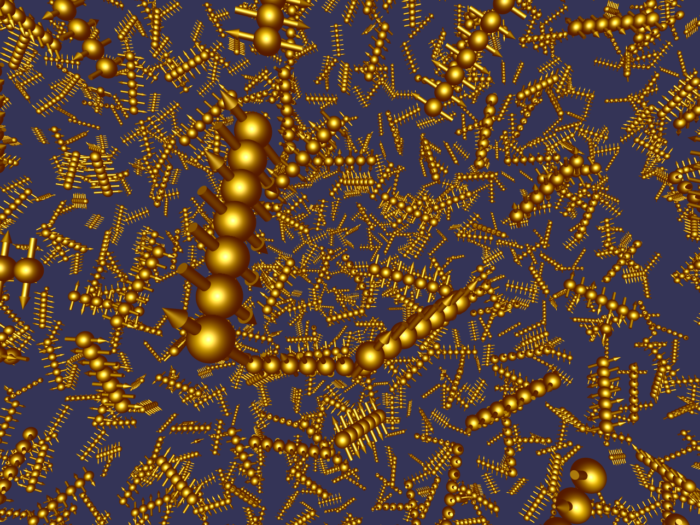# Interaction Distance: A New Way of Studying Strongly Correlated Systems

Quantum many-body systems represent a challenge for theoretical physics due to the complexity of the total wave function describing the system. A quantum many-body state, in general, requires an exponentially large number of parameters to be specified. Furthermore, the presence of entanglement enriches the nature of correlations hosted in such systems in relation to their classical counterparts. For example, a state of a classical spin system can be fully specified by fixing the orientation of each of the spins independently. This is a description of only polynomial cost. In contrast, a state of quantum spin system is a superposition over the incredible multitude of possible classical configurations (see Figure below). It is exactly the famous principle of quantum superposition, together with quantum entanglement, that make the study of quantum many-body systems an immensely complicated affair.Figure: A quantum state of spins can be a superposition of exponentially many classical configurations where each spin points up or down. This makes such states very difficult to understand.

As a consequence, quantum many-body problems rarely permit complete analytical solutions. Because of this, one traditionally resorts to perturbation theory and mean-field theory to obtain approximate solutions to the problem, both in condensed matter and high energy physics. However, a plethora of other interesting and fundamental phenomena arise in systems which are believed to be "far" from a free or non-interacting model. Analysing such systems often requires a variational ansatz, which is restrictive in that it necessitates some non-trivial assumptions about the emergent behaviour (e.g., knowing the nature of the quasiparticles in the context of condensed matter physics or the amount of entanglement in tensor network methods).

Work presented here describes how it is possible to quantify the "freedom" of a many-body ground-state, only by examining its entanglement structure. This is done by identifying the "nearest" free entanglement-structure to the entanglement structure of the ground state of a given interacting system. The measure of such a property is the interaction distance${D_\mathcal{F}}$. In this context, "freedom" has a specific definition to mean the factorability of the entanglement spectrum, which encodes all the quantum correlations present in the many-body state and is obtained as the spectrum of the reduced density matrix,$\rho$, of the ground state. Within the entanglement Hilbert space, where all the entanglement spectra are defined, there is a manifold containing all density matrices that result from reducing a free-fermion system to a subsystem. Thus, in other words,${D_\mathcal{F}}$ is the distance of the reduced density matrix in question to the nearest point on this manifold.

In a physical sense$D_\mathcal{F}$ measures the distinguishability of the optimal free state from the state$\rho$ when measuring observables. We see the interaction distance as an exciting theoretical tool because it can be used on any problem of quantum many-body physics, from systems deemed too complex to explore analytically, to previously solved models which can be re-analysed from a fresh perspective.

In the first paper, Optimal free descriptions of many-body theories, we introduced the notion of interaction distance and explored its general properties. This paper also contains a case study of the transverse Ising model in longitudinal field, for which we identify the optimal free model throughout the phase diagram.

In the subsequent paper we showed how the interaction distance can be used to quantify the distinguishability of topological states of matter from states of free fermions. The paper explored a large class of topological states, including parafermion chains and string-net models.